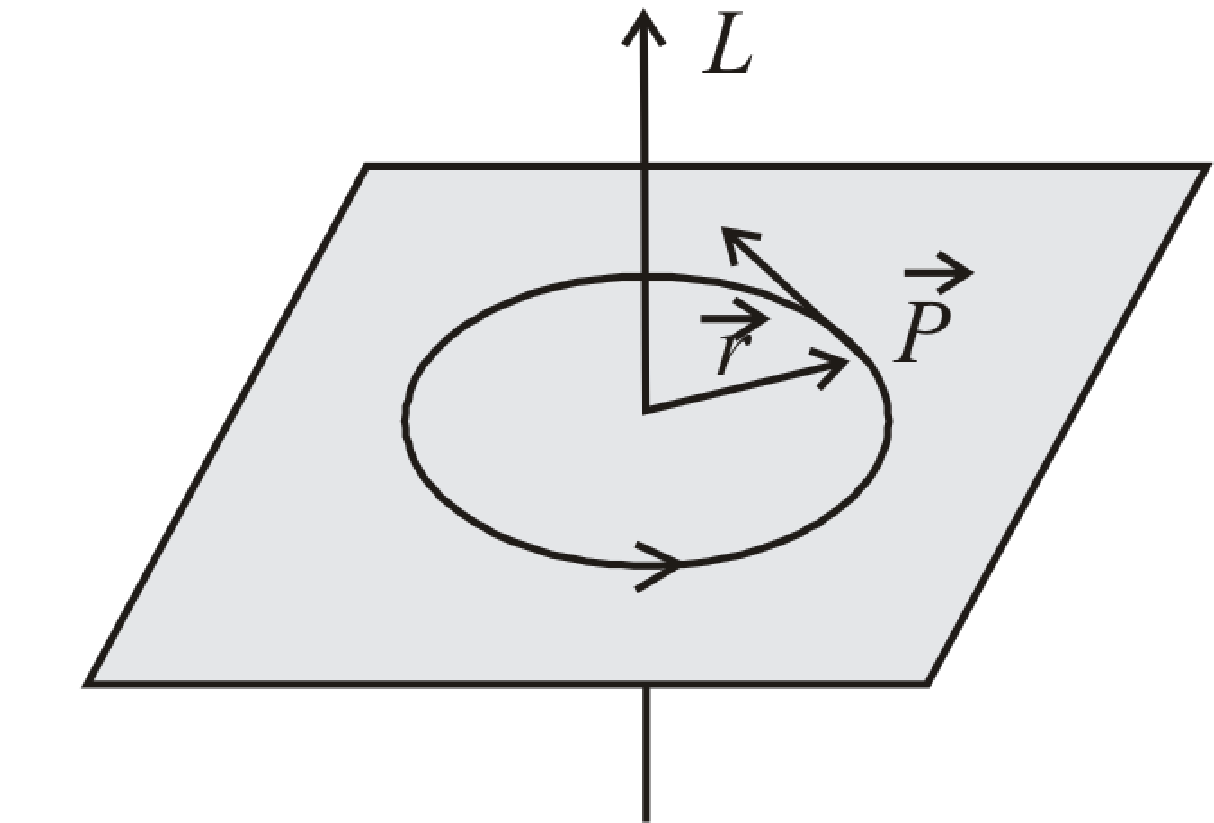BodhiAI
Oct. 31, 2019

#### Angular Momentum:

Angular Momentum :Ifis the linear momentum of particle andis its position vector from the point of rotation then angular momentum.(1) S. I. Unit :kg-m2-s–1or J-sec.

(2) Dimension : [ML2T–1] and it is similar to Planck’s constant (h).

(3) In cartesian co-ordiantes ifandThen=(4) In case of circular motion= mvr sin φ

• L = mvr = mr2ω [Asand v = rω]

orL = Iω [As mr2 = I]

In vector form(5) From∴=[Asand]

(6) If a large torque acts on a particle for a small time then 'angular impulse' of torque is given byOr Angular impulseAngular impulse = Charge in angular momentum

(7) The angular momentum of a system of particles is equal to the vector sum of angular momentum of each particle i.e.,